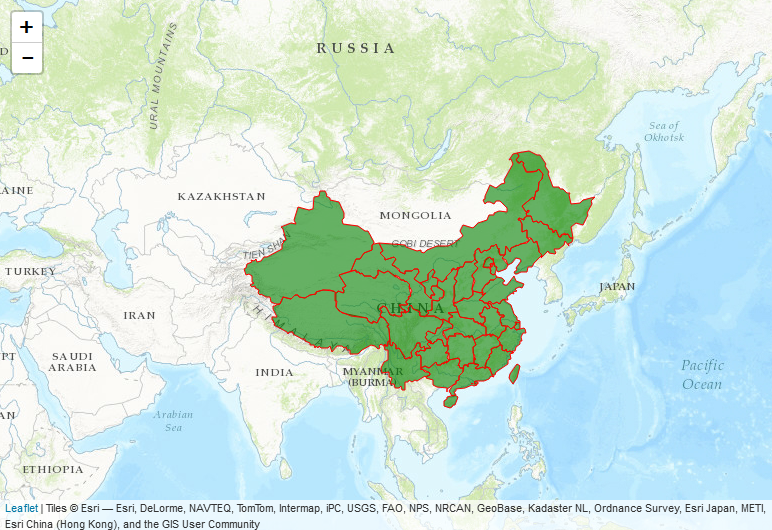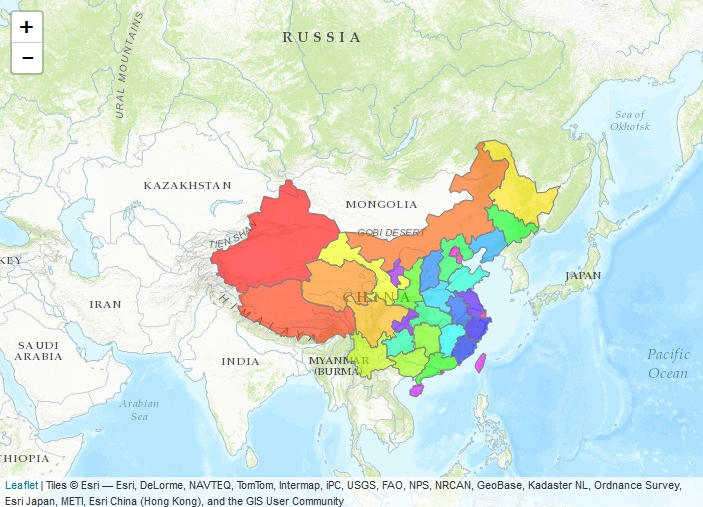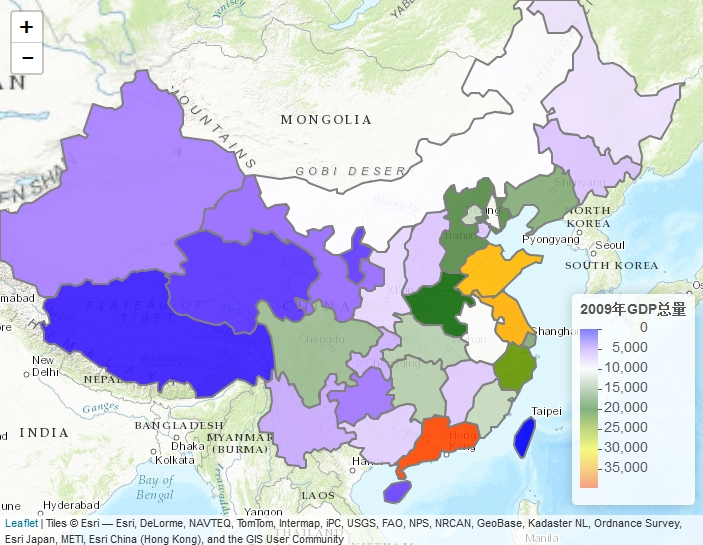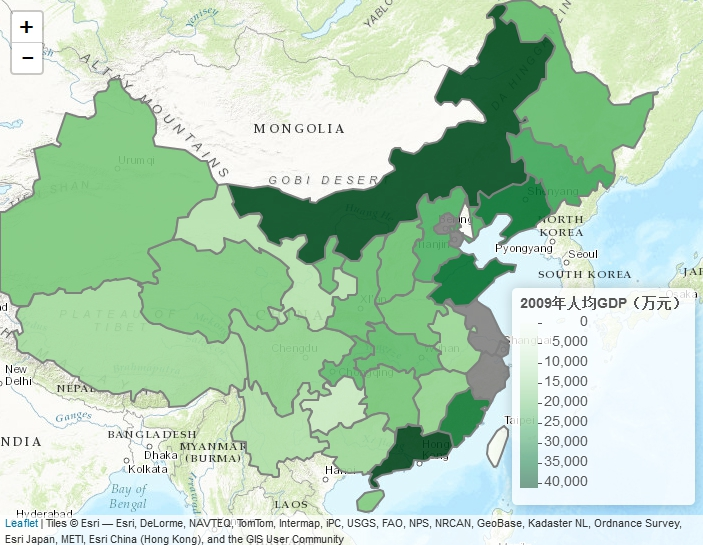Wechat: yu389741| Email: gisdqy@163.com

# R语言在线地图神器：Leaflet for R包（五） GeoJson文件的使用

GeoJSON是一种对各种地理数据结构进行编码的格式，基于Javascript对象表示法的地理空间信息数据交换格式。GeoJSON对象可以表示几何、特征或者特征集合。GeoJSON支持下面几何类型：点、线、面、多点、多线、多面和几何集合。GeoJSON里的特征包含一个几何对象和其他属性，特征集合表示一系列特征。——百度百科

addGeoJSON()

addTopoJSON()

 geoData <- readLines(“e:/china.json”,warn=FALSE) %>%   paste(collapse = “\n”)%>%fromJSON(simplifyVector = FALSE) geoData\$style = list(   weight = 1,   color =”red”,   fillColor=”green”,   opacity = 1,   fill = TRUE,   fillOpacity = 0.6 ) leaflet() %>% setView(lng = 98.583, lat = 39.833, zoom = 3) %>%   addProviderTiles(“Esri.WorldTopoMap”)%>%addGeoJSON(geoData)geoData\$style = list(   weight = 1,   color =”gray”,   opacity = 1,   fill = TRUE,   fillOpacity = 0.6 ) pal <- substr(rainbow(34),1,7) i<-0 geoData\$features <- lapply(geoData\$features, function(feat) {   i<<-i+1   feat\$properties\$style <- list(     fillColor = pal[i]   )   feat }) leaflet() %>% setView(lng = 98.583, lat = 39.833, zoom = 3) %>%   addProviderTiles(“Esri.WorldTopoMap”)%>%addGeoJSON(geoData)library(jsonlite) geoData2 <- readLines(“e:/china_pop_gdp.json”,warn=FALSE) %>%   paste(collapse = “\n”)%>%fromJSON(simplifyVector = FALSE) geoData2\$style = list(   weight = 2,   color =”gray”,   opacity = 1,   fill = TRUE,   fillOpacity = 0.9 ) GDP_2009 <- sapply(geoData2\$features, function(feat) {   feat\$properties\$GDP_2009 })   pal <- colorNumeric(c(“blue”, “white”, “darkgreen”, “yellow”, “orangered”), GDP_2009) geoData2\$features <- lapply(geoData2\$features, function(feat) {   feat\$properties\$style <- list(     fillColor = pal(feat\$properties\$GDP_2009)   )   feat }) leaflet() %>% setView(lng = 98.583, lat = 39.833, zoom = 3) %>%   addProviderTiles(“Esri.WorldTopoMap”)%>%addGeoJSON(geoData2)%>%   addLegend(“bottomright”, pal = pal, values = GDP_2009,title = “2009年GDP总量”)geoData2 <- readLines(“e:/china_pop_gdp.json”,warn=FALSE) %>%   paste(collapse = “\n”)%>%fromJSON(simplifyVector = FALSE) geoData2\$style = list(   weight = 2,   color =”gray”,   opacity = 1,   fill = TRUE,   fillOpacity = 0.9 ) GDP_2009 <- sapply(geoData2\$features, function(feat) {   feat\$properties\$GDP_2009 }) POP_2009 <- sapply(geoData2\$features, function(feat) {   feat\$properties\$Pop_2009 }) #人均GDP专题图 pal <- colorNumeric(“Greens”, (GDP_2009*10000)/max(1,POP_2009)) geoData2\$features <- lapply(geoData2\$features, function(feat) {   feat\$properties\$style <- list(     fillColor = pal((feat\$properties\$GDP_2009*10000)/max(1,feat\$properties\$Pop_2009))   )   feat }) leaflet() %>% setView(lng = 98.583, lat = 39.833, zoom = 3) %>%   addProviderTiles(“Esri.WorldTopoMap”)%>%addGeoJSON(geoData2)%>%   addLegend(“bottomright”, pal = pal,value =(GDP_2009*10000)/(max(1,POP_2009)), title = “2009年人均GDP（万元）”)1、GeoJson需要开销大量的内存，对比同等的空间对象来，它耗的内存，大约在5-8倍左右。所以如果你的节点特别多，那么geojson就会开销非常非常大，带来的结果就是非常非常慢……

2、GeoJson绘制在地图的时候，无法使用弹出窗